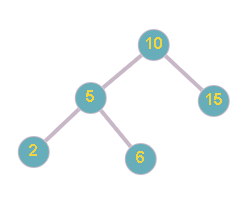# Floor in BST

Posted: 2 Dec, 2020
Difficulty: Moderate

## PROBLEM STATEMENT

#### For example:``````In the above example, For the given BST  and X = 7, the greatest value node of the BST  which is smaller than or equal to  7 is 6.
``````
##### Input Format:
``````The first line of input contains an integer ‘T’, denoting the number of test cases. Then each test case follows.

The first line of each test case contains nodes in level order form (separated by space). If any node does not have a left or right child, take -1 in its place.

The second and the last line of each test case contain integer ‘X’.

For example, the input for the tree depicted in the below image.
````````````10
5 15
2 6 -1 -1
-1 -1 -1 -1
``````
##### Explanation :
``````Level 1 :
The root node of the tree is 10

Level 2 :
Left child of 10 = 5
Right child of 10 = 15

Level 3 :
Left child of 5 = 2
Right child of 5 = 6
Left child of 15 = null(-1)
Right child of 15 =  null (-1)

Level 4 :
Left child of 2 = null (-1)
Right child of 2 = null (-1)
Left child of 6 = null (-1)
Right child of 6 = null (-1)

The first not-null node(of the previous level) is treated as the parent of the first two nodes of the current level. The second not-null node (of the previous level) is treated as the parent node for the next two nodes of the current level and so on.
The input ends when all nodes at the last level are null(-1).
``````
##### Note :
``````The above format was just to provide clarity on how the input is formed for a given tree.
The sequence will be put together in a single line separated by a single space. Hence, for the above-depicted tree, the input will be given as:
10 5 15 2 6 -1 -1 -1 -1 -1 -1
``````
##### Output Format:
``````For each test case, print a single integer ‘M’, denoting the greatest value node of the BST  which is smaller than or equal to  ‘X’.

The Output of each test case will be printed on a separate line.
``````
##### Note:
``````You do not need to print anything, it has already been taken care of. Just implement the given function.
``````
##### Constraints:
``````1 <= T <= 5
1 <= N <= 5 * 10 ^ 3
1 <= nodeVal[i] <= 10 ^ 9

Time Limit: 1 sec.
``````Approach 1

#### Intuition:

• Traverse the tree using any tree traversal technique (Inorder or Preorder or Postorder)
• Keep track of the closest smaller or same element.

#### Approach:

• Either the root element is the key node.
• Key node lies left of root
• Key node lies right of the node.

Here the key node is the node which is closest smaller or same element.

## Algorithm :

Let the ‘floorInBST’ be the function that accepts two parameters (root node of tree(root), given integer(K)). It returns the greatest value node of the BST  which is smaller than or equal to  ‘X’.

Steps are as follow:

• If the root is ‘NULL’ then return -1.
• Take a variable ‘ans’, initialize it to -1.
• Recursively call the function on the left subtree and store the result in a variable say ‘leftFloor’.
• Recursively call the function on the right subtree and store the result in a variable say ‘rightFloor’.
• If right floor is less than 'X' and but greater then left floor
• Update ‘ans’ with ‘rightFloor’.
• If left floor is less than 'X' and but greater then right floor
• Update ‘ans’ with ‘leftFloor’.
• If root -> val is less than 'X' and greater than 'ans' then root value is more close to 'X'
• Update ‘ans’ with root -> val.
• Return ‘ans’.